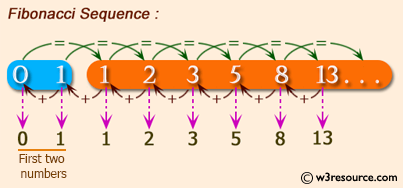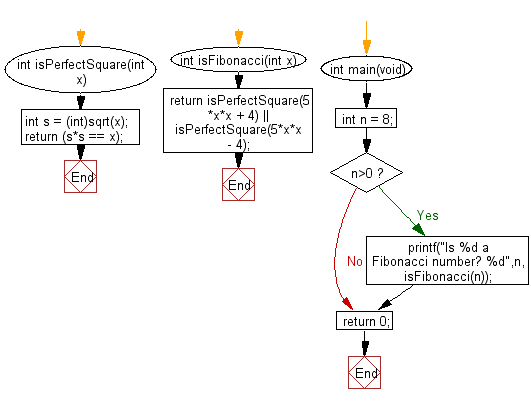﻿ C Program: Find out if a number is Fibonacci

# C Exercises: Check if a given number is Fibonacci number or not

## C Programming Mathematics: Exercise-26 with Solution

Write a C program to check if a given number is a Fibonacci number or not.

In mathematics, the Fibonacci numbers, commonly denoted Fn form a sequence, called the Fibonacci sequence, such that each number is the sum of the two preceding ones, starting from 0 and 1. That is, and for n > 1. By starting with 1 and 2, the first 10 terms will be: 1, 2, 3, 5, 8, 13, 21, 34, 55, 89.

Example:
Input: n = 8
Output: Is 8 a Fibonacci number? 1

Pictorial Presentation:Sample Solution:

C Code:

``````#include <stdio.h>
#include <math.h>
int isPerfectSquare(int x)
{
int s = (int)sqrt(x);
return (s*s == x);
}
int isFibonacci(int x)
{
return isPerfectSquare(5*x*x + 4) ||
isPerfectSquare(5*x*x - 4);
}
int main(void)
{
//int n = 12;
//int n = 55;
int n = 8;
if (n>0)
{
printf("Is %d a Fibonacci number? %d",n, isFibonacci(n));
}
return 0;
}
```
```

Sample Output:

```Is 8 a Fibonacci number? 1
```

Flowchart:C Programming Code Editor:

Improve this sample solution and post your code through Disqus.

What is the difficulty level of this exercise?

Test your Programming skills with w3resource's quiz.

﻿